# Lines of symmetry for regular polygons:

Polygon is a closed figure made of several line segments. A polygon is said to be regular if all its sides are of equal length and all its angles are of equal measure.

1) An equilateral triangle is a regular polygon of three sides. An equilateral triangle is regular because each of its sides has the same length and each of its angles measures 60°.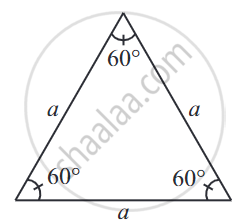2) A square is also regular because all its sides are of equal length and each of its angles is a right angle (i.e., 90°). Its diagonals are seen to be perpendicular bisectors of one another.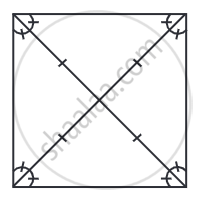3) If a pentagon is regular, naturally, its sides should have equal length. The measure of each of its angles is 108°.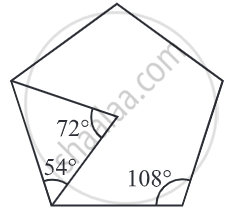4) A regular hexagon has all its sides equal and each of its angles measures 120°.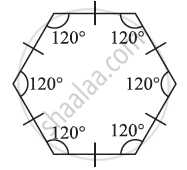The regular polygons are symmetrical figures and hence their lines of symmetry are quite interesting, Each regular polygon has multiple lines of symmetry.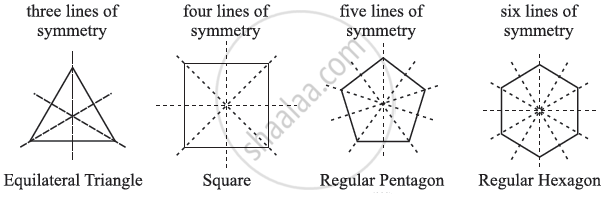If you would like to contribute notes or other learning material, please submit them using the button below.

### Shaalaa.com

Number of Lines of Symmetry of a Circle [00:05:26]
S
0%